# Numerical Simulation of the Impact of Unit Commitment Optimization and Divergence Angle on the Flow Pattern of Forebay

Numerical Simulation of the Impact of Unit Commitment Optimization and Divergence Angle on the Flow Pattern of Forebay

Cundong Xu Hongyang Zhang Xianqi Zhangg Liwei Han Rongrong Wang Qinyu Wen Lianying Din

School of Water Conservancy, North China University of Water Resources and Electric Power/Collaborative Innovation Center of Water Resources Efficient Utilization and Guarantee Engineering, Henan Province, Zhengzhou, China.

Corresponding Author Email:
zhanghongyang@ncwu.edu.cn
Page:
91-96
|
DOI:
https://doi.org/10.18280/ijht.330215
N/A
|
Accepted:
N/A
|
Published:
30 June 2015
| Citation

OPEN ACCESS

Abstract:

The high-lift pumping station that lifts water from sediment-laden rivers presents a serious problem that sediments deposit in forebay. To solve this problem, this paper proposes a three-dimensional numerical simulation method of water flows based on Fluent (a fluid mechanics simulation software). By constructing two models, namely the mathematical model and the turbulence model, this paper conducts numerical simulation on the flow pattern of forebay. According to the simulated velocity contour map, on-site inspection and survey, the paper studies how unit commitment optimization and divergence angle can affect flow pattern and sediment deposition in forebay. Results show that forebay keeps a good flow pattern if the units take turns to operate symmetrically. The forebay will have no reverse current zones and little sediment deposition. As the divergence angle increases between 20°～40°, the flow pattern gets worse and there are more eddy zones. Consequently, reasonable unit commitment optimization and divergence angle have a positive influence on flow pattern in forebay and are able to reduce sediment deposition. These findings can support improvement on large pumping station design and serve as theoretical reference for the operation of large pumping station.

Keywords:

High-lift pumping station; front inflow forebay; three-dimensional flow pattern; numerical.

1. Introduction

The forebay is the intake structure of the pumping station. It keeps the water flow smoothly from the approach channel to the intake sum by supplying water to the water inlet of every unit directly and evenly . The divergence angle of front inflow forebay is the main factor that influences the forebay flow pattern and its scale. Unsuitable geometry size can result in uneven distribution flow velocity in forebay, and produce stagnant water area, reverse current zones and vortex. These further lead to insufficient water flow in some units and sediment deposition in forebay [2-4]. If the vortex brings air into the water pumps, then it will reduce the efficiency of the water pumps greatly, lead to their cavitation and unit vibration, or even stop them from sucking water [5-7].

Jingtaichuan pumping irrigation project in Gansu province, China (hereinafter “Jingdian project”) has 43 large cascade pumping stations. Water comes from the Yangtze River which has high content of sands during flood season, thus the unsuitable design and operation mode lead to serious problem of sediment deposition in forebay. In some pumping stations, the sediments account for more than a half of the forebay’s total area, which affects the intake flow pattern and efficiency of the pumping station .

Figure 1 is a typical pumping station to show sediment in forebay.

## 1.jpg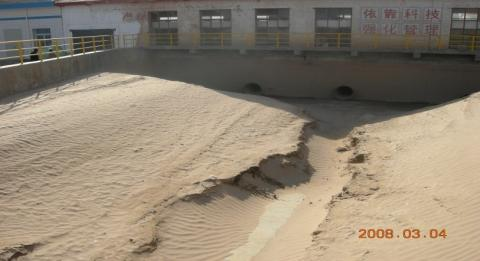Figure 1. Typical conditions of sediment in forebay

In order to achieve preferable forebay flow pattern, hydraulic model tests were often used to conduct research on the layout, scale, modification of flow pattern, sediment deposition prevention and operation mode before many large pumping stations were designed [9-11]. With the successful application of numerical simulation technology to hydromechanics, such technology helps with visual and precise analysis of the flow pattern, thus provides a theoretical basis and technology support for the optimal design [12-14].

In order to optimize the thigh-lift pumping station of Jingdian project, this paper takes Fluent [15-16] as a platform, employs three-dimensional numerical simulation method, builds mathematical model and turbulence model and conducts numerical simulation on the flow pattern of forebay. Results show that the numerical simulation fits well with that of the model test and on-site monitoring, suggesting that this method has sufficient reliability to simulate the flow pattern of pumping station forebay.

2. Mathematical Model

2.1 Governing equation

For incompressible pumping station forebay flow in steady conditions, the governing equation is:

Equation of continuity:

$\frac{\partial u_{i}}{\partial x_{i}}=0$    (1)

Equation of momentum:

$\frac{\partial}{\partial t}\left(\rho u_{i}\right)+\frac{\partial}{\partial x_{j}}\left(\rho u_{i} u_{j}\right)=-\frac{\partial \rho}{\partial x_{i}}+\frac{\partial}{\partial x_{j}}\left(\mu \frac{\partial u_{i}}{\partial x_{j}}-\rho \overline{u_{i}^{\prime} u_{j}^{\prime}}\right)+S_{i}$   (2)

In the equation, $u_{i}$, $u_{j}$ is the flow velocity in each direction; $x_{i}$, $x_{j}$ is coordinate axis; $S_{i}$ is the source term; subscript i, j can be set as 1, 2, 3 to describe space coordinate; $-\rho \overline{u_{i}^{\prime} u_{j}^{\prime}}$ is reynolds stress, $\tau_{i j}=-\rho \overline{u_{i}^{\prime} u_{j}^{\prime}}$.

2.2 Standard $k-\varepsilon$ turbulence model

Equation of turbulence kinetic energy is:

$\frac{\partial(\rho k)}{\partial t}+\frac{\partial\left(\rho \varepsilon u_{i}\right)}{\partial x_{i}}=\frac{\partial}{\partial x_{j}}\left[\left(\mu+\frac{\mu_{t}}{\sigma_{\varepsilon}}\right) \frac{\partial k}{\partial x_{j}}\right]+G_{k}-\rho \varepsilon$   (3)

$\frac{\partial(\rho \varepsilon)}{\partial t}+\frac{\partial\left(\rho \varepsilon u_{i}\right)}{\partial x_{i}}=\frac{\partial}{\partial x_{j}}\left[\left(\mu+\frac{\mu_{t}}{\sigma_{\varepsilon}}\right) \frac{\partial k}{\partial x_{j}}\right]+C_{1 \varepsilon} \frac{\varepsilon}{k} G_{k}-C_{2 \varepsilon} \rho \frac{\varepsilon^{2}}{k}$   (4)

In the equation, $G_{k}$ is the production term of turbulence kinetic energy $k$, $G_{k}=\mu_{t}\left(\frac{\partial u_{i}}{\partial x_{j}}+\frac{\partial u_{j}}{\partial x_{i}}\right) \frac{\partial u_{i}}{\partial x_{j}}$ , $\mu_{t}=\rho C_{u} \frac{k^{2}}{\varepsilon}$; empirical coefficient $C_{1 \varepsilon}$=1.44, $C_{2 \varepsilon}$=1.92, $C_{3 \varepsilon}$=0.09, $\sigma_{\varepsilon}$ =1.3, $\sigma_{k}$ =1.0.

2.3 Boundary conditions

(1) Inlet boundary conditions: the flow at the inlet section is fully developed turbulence. The average flow velocity at the inlet section can be known from the design flow of the pumping station and the size of the outlet section. Flow velocity distribution is set as the boundary condition.

(2) Outlet boundary conditions: As the flow velocity and pressure of the outlet is unknown before working out the equation and under the assumption that the flow at the outlet is free, free outflow is taken as the flow condition at the outlet.

(3) Non-slip wall condition: Turbulence model is only effective when there is fully developed turbulent. While in the wall area, the flow is changeable. So when the turbulence model is used to describe turbulent and simulate velocity field that has sidewalls, the sidewalls should be subject to wall-function method.

(4) Free surface: The surface of the forebay is free surface. The velocity of the free face and turbulence kinetic energy can be seen as symmetrical plane.

3. Calculation Object

Xinqianer pumping station of the Jingdian project is taken as an example, with flat structure shown in Figure 2. 1, 2, 3, 4, 5, 6 represent the numbers of the pumping station pumping units respectively. The pipe diameter of 1, 3, 5 and 6 is 800mm and the flow rate is 0.8m3/s. The pipe diameter of 2, 4 is 1000mm and the flow rate is 1.53m3/s. The design flow of the upstream cannel is 6.87m3/s and the increase-flow is 8.12m3/s.

## 2.pngFigure 2. Plan of the Xinqianer pumping station of the Jingdian project   α=24°

4. Simulation Result and Analysis

4.1 Heat conduction equation

Figgure 2~5 are the velocity contour map and velocity vector streamline diagram of the simulated forebay of different unit commitment. In the velocity contour map, the red is the maxima velocity while the blue is the minimum one. In other word, the velocity decreases from the red to the blue. The figures show clearly that the velocity reaches the peak when it flows to the forebay and starts to decrease when water passes the transition sector. Because of the pier, the flow velocity is further reduced when the water flows to the trashrack, as is shown in the green part of the figure. When water passes the diffusion sector and comes to the inlet of the water pumps, the flow velocity reaches the lowest, as is showed in the blue part of the figure.

We can see from the velocity contour map that the flow velocity is almost zero at the reverse current zones. In the reverse current zones, the silt tends to deposit into sediment deposition because of the sharp decrease of flow velocity. The velocity vector streamline diagram shows that the flow pattern is preferable in a symmetrical and steady way and the silt is hard to deposit in the forebay when all units operate symmetrically at intervals. While when the units don’t run symmetrically, there are obvious reverse current zones and can easily form sediment deposition.

## 3a.png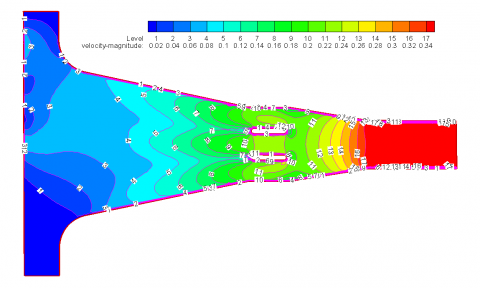(a) Velocity contour map

## 3b.png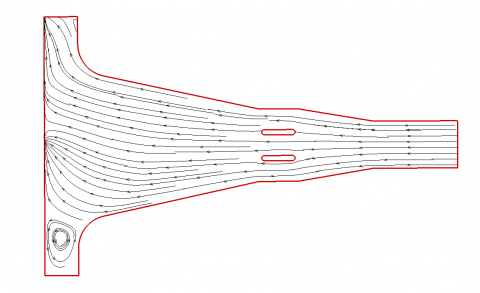(b) Velocity vector streamline diagram

Figure 3. Forebay flow pattern when 1, 2, 3 units are on

## 4a.png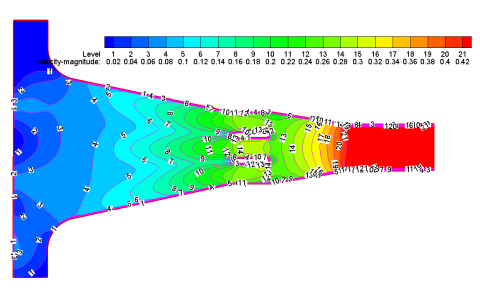(a) Velocity contour map

## 4b.png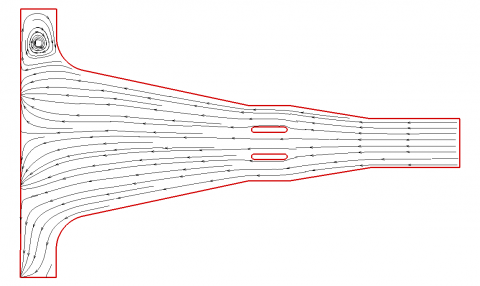(b) Velocity vector streamline diagram

Figure 4. Forebay flow pattern when 2, 4, 6 units are on

## 5a.png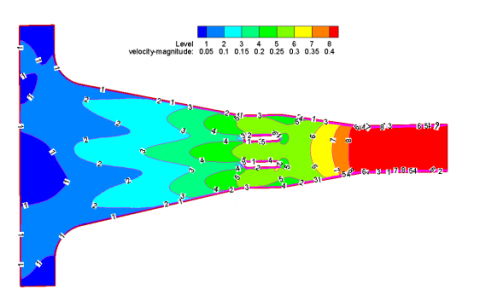(a) Velocity contour map

## 5b.png(b) Velocity vector streamline diagram

Figure 5. Forebay flow pattern when 1, 2, 5, 6 units are on

## 6a.png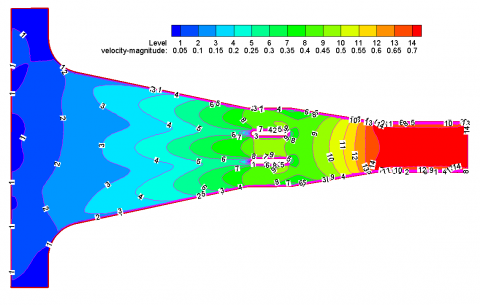(a) Velocity contour map

## 6b.png(b) Velocity vector streamline diagram

Figure 6. Forebay flow pattern when all units are on

4.2 The influence of forebay divergence angle on forebay flow pattern

The watered-out direction of the front inflow forebay fits the inflow direction of the pump sump. It features simple pattern, convenient construction and steady flow. Limited by the natural divergence angle of the flow, the divergence angle of the pumping station forebay shouldn’t be too large. The length of the forebay needs to be extended if there are many pumping units, which will add extra engineering work. Thus, it is important to shorten the forebay while making sure the flow pattern and the divergence angleα are appropriate. The divergence angle of front inflow forebay should be 20°～40° at current design specifications. The divergence angle of the

pumping station front inflow forebay in Jingdian project is usually 30°≥α≥20°. So α is set as 20°, 24°, 30° and unit 1 is opened to do simultation.

Figure 7~9 are the forebay velocity contour map and velocity vector streamline diagram when α is set as 20°, 24°, 30° and Xiganer pumping station unit 1 is on. The simulation shows that the other side of the forebay has vortex under such operation mode; the eddy zones expands as the divergence angle increases. Such bad pattern can lead to sediment deposition in forebay. At the meantime, once there is air-intake funnel vortex in these eddy zones, air will enter the water pumps together with water flow, which will lower the efficiency of the water pumps and create serious vibration in the water pumps.

Divergence angle at 20°:

## 7a.png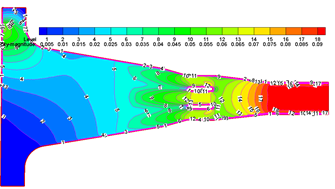(a) Velocity contour map

## 7b.png(b) Velocity vector streamline diagram

Figure 7. Forebay flow pattern when unit 1 is on

Divergence angle at 24°:

## 8a.png(a) Velocity contour map

## 8b.png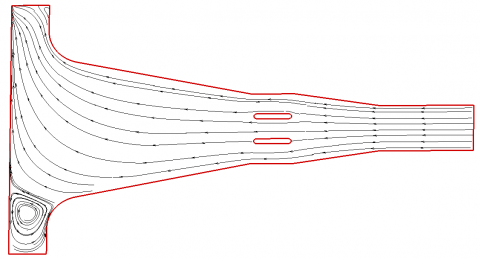(b) Velocity vector streamline diagram

Figure 8. Forebay flow pattern when unit 1 is on

Divergence angle at 30°:

## 9a.png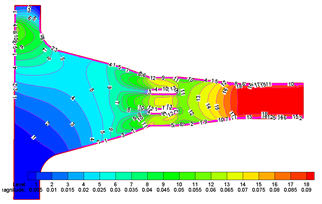(a) Velocity contour map

## 9b.png(b) Velocity vector streamline diagram

Figure 9. Forebay flow pattern when unit 1 is on

5. Conclusion

A bad flow pattern in the pumping station forebay would cause a large amount of sediment deposition. It would also reduce pumping efficiency of water pumps. To solve this problem, this paper proposes a three-dimensional numerical simulation method of water flows based on Fluent. Simulation results and on-site inspection show that the simulated flow pattern fits well the flow pattern in practice.  Numerical simulation shows that the flow pattern of pumping station forebay is affected by the followings: Firstly, the forebay structure. It would affect the flow pattern by influencing flow direction and flow velocity of water. Secondly, the unit commitment. If only one side of unit commitment opens, the other side would produce reverse currents and eddy zones. If the units take turn to open symmetrically, reverse currents are not likely to occur in forebay. In this way, there is little sediment deposition. Thirdly, sediment deposition would change the flow pattern in forebay. As sediments occupy some space, the structure of forebay is changed and the water cannot flow as designed. This leads to lower pumping efficiency, which makes sediment deposition in forebay even worse.

Acknowledgement

This work was financially supported by the “Natural Science Foundation of China (51279064; 31360204)”, the “Supported by Program for Science & Technology Innovation Talents in Universities of Henan Province (14HASTIT047)” and the “Supported by Program for Innovative Research Team (in Science and Technology) in University of Henan Province (14IRTSTHN028)”.

References

1 Luo Can, Cheng Li, Liu Chao. Numerical simulation of mechanism for sill rectifying flow in pumping station intake [J]. Journal of Drainage and Irrigation Machinery Engineering, 2014, 32(5): 393-398.

2 Wang Wuchang, Wang Fujun, Yu Xin, et al. Application of Lbm-Sgs model to flows in a pumping

Station forebay [J]. Journal of Hydrodynamics, 2010, 22(2): 196-206.

3 Wang Fangfang, Wu Shiqiang, Xiao Xiao, et al. 3-D numerical simulation for side-inlet forebay of a pumping station [J]. Hydro-science and Engineering, 2014, 2:54-59.

4 Li Xiaoxu, Zheng Yuan, Mao Yuanting, et al. Study of automatic tracking system of solar locator based on seven-point method [J]. Water Resources and Power, 2011, 29(7): 132-135+145.

5 Guo XiaoMei, Zhu Linhang, Zhu ZuChao, et al. Numerical and experimental investigations on the cavitation characteristics of a high-speed centrifugal pump with a splitter-blade inducer [J]. Journal of Mechanical Science and Technology, 2015, 29(1): 259-267.

6 Gao Bingbiao, Wang Qiang, Zhang Jianjun, et al. Occurrence mechanism and countermeasures of pump cavitation [J]. Agricultural Equipment & Vehicle Engineering, 2013, 51(2): 53-54+73.

7 Ba Duoduo, Ma Tailing. Water pump and water pump station [M]. Beijing: China WaterPower Press, 2004.

8 Xu Cundong, Cui Xiaoyan, Wang Xiaozhen. Research on the reasons of the sediment deposition and the measures of anti-sedimentation in the side-direction inflow forebay of pumping station [J]. New Technology & New Process, 2010, 10:5-8.

9 Wang Junxing, Chen Xiaoyong, Yin Hao, et al. Study on the hydraulic model tests of Hunanzhen hydropower station [J]. Journal of Hydroelectric Engineering, 2008, 27(5): 103-108.

10 Feng Jiangang, Li Xin. Research on the measures of improve the flow pattern of the forebay of city intake water pumping stations [J]. Applied Mechanics and Materials, 2012,170-173: 1856-1861.

11 Chen Fangni, Shen Ying, Bai Yuchuan. Experiment on physical model of intake at pumping station [J]. Journal of Water Resources and Water Engineering, 2013, 24(2): 155-159.

12 Li Jun, Cao Yongmei, Gao Chuanchang. Numerical simulation of flow patterns in the forebay and suction sump of tianshan pumping station [J]. Water Practice and Technology, 2014, 9(4): 519-525.

13 Wu Jianhuang, Liu Guiying, Huang Wenhua, et al. Transient blood flow in elastic coronary arteries with varying degrees of stenosis and dilatations: CFD modelling and parametric study [J]. Computer Methods in Biomechanics and Biomedical Engineering, 2015,18(16): 1835-1845.

14 Keshmiri Amir, Uribe Juan, Shokri Nima. Benchmarking of Three Different CFD Codes in Simulating Natural, Forced, and Mixed Convection Flows [J]. Numerical Heat Transfer Part A-Applications, 2015, 67(12): 1324-1351.

15 Kuang, Weihua, Pang, Xufeng. Flow Characteristics

Study of Transit Time Ultrasonic Flowmeter Based on Fluent [J]. Advances in Information Sciences and Service Sciences, 2012, 4(21): 413-421.

16 Duan Yulei, Zhu Jinbo, Wang Mengmeng, et al.Gap between impeller and cover plate Fluent Fluid Dynamics validation [J]. Coal Mine Machinery, 2013, 34(3): 75-76.